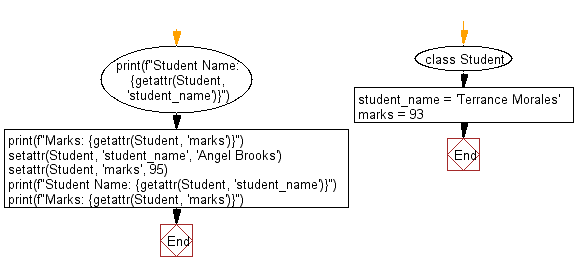﻿ Python: Modify the attribute values of a given class - w3resource# Python: Modify the attribute values of a given class

## Python Class ( Basic ): Exercise-9 with Solution

Write a Python class named Student with two attributes student_name, marks. Modify the attribute values of the said class and print the original and modified values of the said attributes.

Sample Solution:

Python Code:

``````class Student:
student_name = 'Terrance Morales'
marks = 93
print(f"Student Name: {getattr(Student, 'student_name')}")
print(f"Marks: {getattr(Student, 'marks')}")
setattr(Student, 'student_name', 'Angel Brooks')
setattr(Student, 'marks', 95)
print(f"Student Name: {getattr(Student, 'student_name')}")
print(f"Marks: {getattr(Student, 'marks')}")
```
```

Sample Output:

```Student Name: Terrance Morales
Marks: 93
Student Name: Angel Brooks
Marks: 95
```

Flowchart:## Visualize Python code execution:

The following tool visualize what the computer is doing step-by-step as it executes the said program:

Python Code Editor:

What is the difficulty level of this exercise?

Test your Programming skills with w3resource's quiz.

﻿

## Python: Tips of the Day

The Zip() Function:

```>>> students = ('John', 'Mary', 'Mike')
>>> ages = (15, 17, 16)
>>> scores = (90, 88, 82, 17, 14)
>>> for student, age, score in zip(students, ages, scores):
...     print(f'{student}, age: {age}, score: {score}')
...
John, age: 15, score: 90
Mary, age: 17, score: 88
Mike, age: 16, score: 82
>>> zipped = zip(students, ages, scores)
>>> a, b, c = zip(*zipped)
>>> print(b)
(15, 17, 16)
```